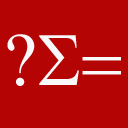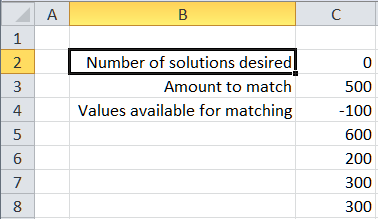Home > Excel > Templates > Match set elementsShare Your

# Find a set of amounts that match a target value

There are instances when one wants to figure out which elements of a set of numbers (amounts) total to a target value.  One instance where this may be necessary is for a company that receives a check for outstanding accounts receivable (A/R) bills but doesn't have matching documentation indicating what bills are being paid.

ReferencesFor a shareware product that addresses the same issue, please visit TM Match Target.

Dean on June 24, 2012

Incredibly useful. Many many thanks.

Doug S on Jan 5, 2012:

Below are the numbers that did NOT work with old method.  It now works!

It seems like each solution is unique such that there is no overlap between each solution.  That's good for me.

It also seems to have fixed some of the bad rounding errors that occurred before such that the target would be 0, but the solution would yield 5.3e-12.  It's really looking good!  Amazing job!

## Excel template using Solver

The Excel template uses Solver to provide one possible combination of numbers that total to the target amount.  Note that like most numerical optimizations, it provides one possible solution.  Others may exist and might be found with a different starting point.  In addition, the template has undergone only basic testing and may or may not be suitable for your specific problem.  Finally, both Solver and the underlying methodology that it employees have inherent limitations in their capabilities.  Some of the limitations, especially in terms of the size of the problem that can be solved, are imposed by Frontline Systems, the producer of the Solver add-in.  It's how it hopes to sell commercial versions of the program, thereby subsidizing the free version that is included with Microsoft Excel.

## VBA code to find multiple matching combinations

There have been several requests for solutions that provide a list of all possible matches.  From the perspective of practicality, one should keep in mind that listing a handful of combinations may allow one to pick the most appropriate or the most meaningful (in the context of one's work).  However, the human mind is unlikely to work well when presented with a lot of valid combinations.

Also, the code may take a fair amount of time to run depending on the size of the list of numbers being searched.

### How to use the code

The data for the search should be organized in a single contiguous range in one column.

The first cell contains the maximum number of solutions to be found.  If the cell contains zero all solutions will be found.

The second cell contains the target value.

The values that are to be matched follow.

Select the range containing all the information (the cell indicating the maximum number of solutions, the target value, and all the values to be matched), and use ALT+F8 to run the startSearch procedure.To improve performance, consider the following issues:

If there are negative values in the amounts to be matched, put them first.

For positive numbers, the larger numbers should be first, i.e., sort the positive numbers in descending order.

### The code

As far as I can tell the code below is easy to understand and is also probably about as fast as one can get.  Put the code below in a standard module.

```Option Explicit

Function RealEqual(A, B, Optional Epsilon As Double = 0.00000001)
RealEqual = Abs(A - B) <= Epsilon
End Function
Function ExtendRslt(CurrRslt, NewVal, Separator)
If CurrRslt = "" Then ExtendRslt = NewVal _
Else ExtendRslt = CurrRslt & Separator & NewVal
End Function
Sub recursiveMatch(ByVal MaxSoln As Integer, ByVal TargetVal, InArr(), _
ByVal HaveRandomNegatives As Boolean, _
ByVal CurrIdx As Integer, _
ByVal CurrTotal, ByVal Epsilon As Double, _
ByRef Rslt(), ByVal CurrRslt As String, ByVal Separator As String)
Dim I As Integer
For I = CurrIdx To UBound(InArr)
If RealEqual(CurrTotal + InArr(I), TargetVal, Epsilon) Then
Rslt(UBound(Rslt)) = (CurrTotal + InArr(I)) _
& Separator & Format(Now(), "hh:mm:ss") _
& Separator & ExtendRslt(CurrRslt, I, Separator)
If MaxSoln = 0 Then
If UBound(Rslt) Mod 100 = 0 Then Debug.Print "Rslt(" & UBound(Rslt) & ")=" & Rslt(UBound(Rslt))
Else
If UBound(Rslt) >= MaxSoln Then Exit Sub
End If
ReDim Preserve Rslt(UBound(Rslt) + 1)
ElseIf IIf(HaveRandomNegatives, False, CurrTotal + InArr(I) > TargetVal + Epsilon) Then
ElseIf CurrIdx < UBound(InArr) Then
recursiveMatch MaxSoln, TargetVal, InArr(), HaveRandomNegatives, _
I + 1, _
CurrTotal + InArr(I), Epsilon, Rslt(), _
ExtendRslt(CurrRslt, I, Separator), _
Separator
If MaxSoln <> 0 Then If UBound(Rslt) >= MaxSoln Then Exit Sub
Else
'we've run out of possible elements and we _
still don't have a match
End If
Next I
End Sub
Function ArrLen(Arr()) As Integer
On Error Resume Next
ArrLen = UBound(Arr) - LBound(Arr) + 1
End Function
Function checkRandomNegatives(Arr) As Boolean
Dim I As Long
I = LBound(Arr)
Do While Arr(I) < 0 And I < UBound(Arr): I = I + 1: Loop
If I = UBound(Arr) Then Exit Function
Do While Arr(I) >= 0 And I < UBound(Arr): I = I + 1: Loop
checkRandomNegatives = Arr(I) < 0
End Function
Sub startSearch()
'The selection should be a single contiguous range in a single column. _
The first cell indicates the number of solutions wanted.  Specify zero for all. _
The 2nd cell is the target value. _
The rest of the cells are the values available for matching. _
The output is in the column adjacent to the one containing the input data.

If Not TypeOf Selection Is Range Then GoTo ErrXIT
If Selection.Areas.Count > 1 Or Selection.Columns.Count > 1 Then GoTo ErrXIT
If Selection.Rows.Count < 3 Then GoTo ErrXIT

Dim TargetVal, Rslt(), InArr(), StartTime As Date, MaxSoln As Integer, _
HaveRandomNegatives As Boolean
StartTime = Now()
MaxSoln = Selection.Cells(1).Value
TargetVal = Selection.Cells(2).Value
InArr = Application.WorksheetFunction.Transpose( _
Selection.Offset(2, 0).Resize(Selection.Rows.Count - 2).Value)
HaveRandomNegatives = checkRandomNegatives(InArr)
If Not HaveRandomNegatives Then
ElseIf MsgBox("At least 1 negative number is present between positive numbers" _
& vbNewLine _
& "It may take a lot longer to search for matches." & vbNewLine _
& "OK to continue else Cancel", vbOKCancel) = vbCancel Then
Exit Sub
End If
ReDim Rslt(0)
recursiveMatch MaxSoln, TargetVal, InArr, HaveRandomNegatives, _
LBound(InArr), 0, 0.00000001, _
Rslt, "", ", "
Rslt(UBound(Rslt)) = Format(Now, "hh:mm:ss")
ReDim Preserve Rslt(UBound(Rslt) + 1)
Rslt(UBound(Rslt)) = Format(StartTime, "hh:mm:ss")
Selection.Offset(0, 1).Resize(ArrLen(Rslt), 1).Value = _
Application.WorksheetFunction.Transpose(Rslt)
Exit Sub
ErrXIT:
MsgBox "Please select cells in a single column before using this macro" & vbNewLine _
& "The selection should be a single contiguous range in a single column." & vbNewLine _
& "The first cell indicates the number of solutions wanted.  Specify zero for all." & vbNewLine _
& "The 2nd cell is the target value." & vbNewLine _
& "The rest of the cells are the values available for matching." & vbNewLine _
& "The output is in the column adjacent to the one containing the input data."
End Sub
```

## References

One can find requests for this kind of help by searching the Google archives of the Excel newsgroups as in http://groups.google.com/groups?as_q=match+numbers+sum+target&as_ugroup=*Excel*  Also, there was a 'challenge' on the mrexcel.com website on this subject some years back but I cannot vouch for the quality of the solution.  The editor claims that there were 3,000+ valid combinations but the code above found 4,000+ after about 15 minutes of searching and it was far from done.

Sum values to target; combination of numbers that equal a value or a given sum; which numbers sum to target